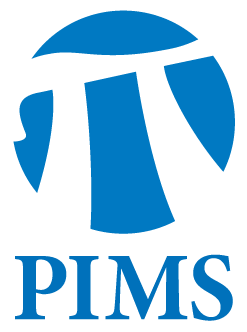## PIMS/AMI Seminar: Yu. M. Kabanov

• Date: 04/12/2012
• Time: 15:30
Lecturer(s):

Yu. M. Kabanov

(Laboratoire de Mathématiques Université de Franche-Comté Besançon, France)

Location:

University of Alberta

Topic:

“Arbitrage Theory for Markets with Transaction Costs”

Description:

Abstract

Classical Arbitrage Theory for frictionless financial markets relates economically meaningful property of absence of arbitrage with the fundamental probabilistic concept of equivalent martingale measure.  Densities processes of equivalent martingale measures plays a role of stochastic deflators.  To compare the present values of assets with their future values one needs to use not the prices but the prices multiplied by stochastic deflators.  The theory of markets with proportional transaction costs treats portfolios as vectors of assets without assigning to them a scalar - its monetary value.  It happens that in the case of proportional transaction costs the fundamental concept is a consistent price system, a martingale evolving in the dual to the solvency cones (in physical units).  In the absence of friction all such martingales can be obtained by multiplying prices by stochastic deflators.   For markets with transaction costs where are several possible formalizations of absence of arbitrage and the available criteria involves consistent price systems. Surprisingly, the passage from the model with a finite number of states of the nature to the general case goes not so smoothly as in the classical theory. Several examples will be discussed in the lecture.  From mathematical point of view, the theory for market with transaction costs is a vector analog of the classical theory. It is a blend of finite dimensional geometry, geometric functional analysis and stochastic calculus.   On the other hand, it feels the gap between mathematical finance and mathematical economics showing how these two disciplines are related.

Other Information:

Refreshments will be served in CAB 649 at 3:00 p.m.

Location: CAB 657